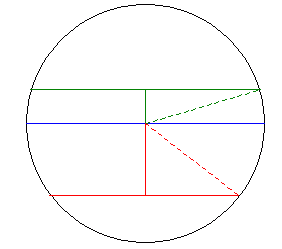Question from Jim: If I know the length of a chord and its distance from the diameter, how do I calculate the radius of the circle? Hi Jim. I've drawn two such chords on the same circle, one red and one green. Then I've drawn a diagonal radius from the end of each chord to the center of the circle, creating right triangles.Can you see why both diagonals are radii and in either case, you can determine the length by using the Pythagorean theorem?   Hope this helps, Stephen La Rocque.